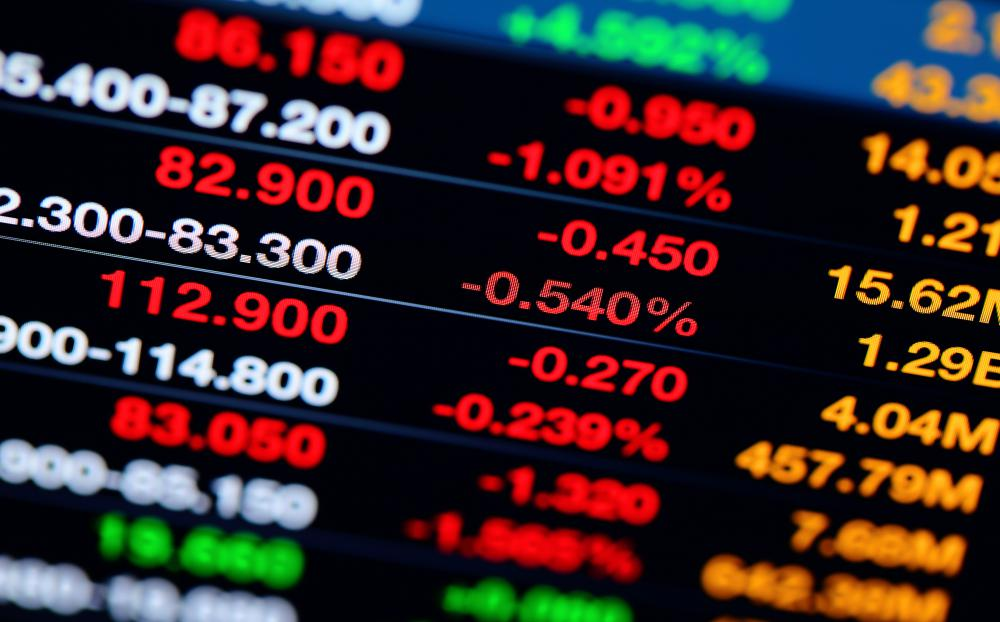# What Is the Role of Moving Averages in Technical Analysis?

A. Garrett

Moving averages in technical analysis help securities traders and others in the finance management industry identify trends related to price changes of stocks and commodities in the open market. There are three types of moving averages in technical analysis: Simple Moving Average (SMA), Linear Weighted Average (LWA) and Exponential Moving Average (EMA). Moving averages are some of the most frequently used technical indicators used in security analysis — technical indicators are statistics derived from market data used to predict shifts in financial assets or economies. Each average is calculated based on the closing price of a security or other financial instrument over a certain period of time. Financial analysts then plot the averages on a chart or graph and look for price trends based on fluctuations in the plot points.Technical analysis indicators are formulas derived from market data such as stock price and the volume of shares being sold over a certain period of time.

SMA is calculated based on the average price of a stock or commodity over a given period of time. It is constantly "moving" because as new closing prices become available, the oldest closing price is dropped. For example, the first day of a five-day moving average is based on the last five closing prices of the security. Each day, a new closing value is added, the oldest closing price is dropped, and a new five day average is calculated. Moving averages in technical analysis of this type give an overview of how a stock or commodity is priced relative to the period of time used.

One criticism of SMA is the fact that each closing price has equal weighting in determining the moving average. In reality, the most recent prices should have a higher weighting because they are the most indicative of future trends. LWA and EMA are two moving averages that were developed to compensate for this discrepancy.

Linear Weighted Average is calculated to reflect the importance of recent prices. Each closing price is multiplied based its position in the data field. For example, when calculating a five-day average, the most recent closing price would be multiplied by five, the second most recent would be multiplied by four, etc. Those values would then be added together and divided by the sum of the multipliers. In this case, the sum of the multipliers would be 15 (5+4+3+2+1=15).

Exponential Moving Average is the most complicated of the moving averages in technical analysis. It is based on a convoluted equation involving SMA, the current price of the security, a smoothing factor to account for price fluctuations, and the number of time periods. Fortunately, most quantitative analysis software packages and spreadsheets have the ability to calculate this average for traders. EMA is sensitive to new data input and consequently provides a more accurate forecast of price changes than SMA.

Once these moving averages in technical analysis are calculated, they are graphed onto charts. A chart showing an upward sloping moving average coupled with a price that is above the moving average indicates an upward trend for a stock or commodity. Alternatively, a downward sloping moving average combined with a price below the moving average indicates a downward trend and usually prompts traders to sell.

Traders may also plot short-term moving averages and long-term moving averages on the same graph — e.g., a chart containing the five day moving average and the 10 day moving average of a stock. If the plot points of the short-term moving average are higher than those of the long-term moving average, the stock's prices are showing an upward trend. Conversely, a short-term moving average that is less than the long-term moving average may indicate a downward trend.

## You might also Like